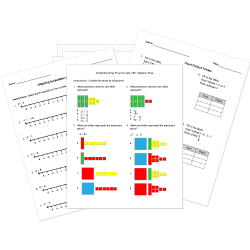Looking for Algebra worksheets?
Check out our pre-made Algebra worksheets!
 Tweet##### Browse Questions
• Arts (209)
• English Language Arts (2742)
• English as a Second Language ESL (1382)
• Health and Medicine (396)
• Life Skills (593)
• Math (2310)

• ### Vectors

• #### Trigonometry

• Physical Education (200)
• Science (3609)
• Social Studies (1794)
• Study Skills and Strategies (15)
• Technology (549)
• Vocational Education (648)

You can create printable tests and worksheets from these Grade 11 Quadratic Equations and Expressions questions! Select one or more questions using the checkboxes above each question. Then click the add selected questions to a test button before moving to another page.

Previous Next
What is the simplified form of $(3x^3y^-4)^-2?$
Exponent answers must have positive exponents in them.
1. $y^8/(6x^-2)$
2. $(9y^8)/(x^6)$
3. $(y^8)/(9x^6)$
4. $(y^8)/(9x^-6)$
Which is standard form of a quadratic function?
1. $y = mx+b$
2. $y = x^3$
3. $y= ax^2 + bx + c$
4. $y=x^2$
Factor the quadratic trinomial $x^2-29x+100$.
1. $(x+25)(x-4)$
2. $(x+25)(x+4)$
3. $(x-25)(x-4)$
4. $(x-25)(x+4)$
Factor the quadratic trinomial $x^2+19x+70$.
1. $(x-14)(x-5)$
2. $(x-15)(x-4)$
3. $(x+15)(x+4)$
4. $(x+14)(x+5)$
What is the factored form for the quadratic trinomial $x^2+8x+15$?
1. $(x-3)(x-5)$
2. $(x+3)(x+5)$
3. $(x-3)(x+5)$
4. $(x+3)(x-5)$
Factor the quadratic trinomial $w^2-8w-84$.
1. $(w-14)(w-6)$
2. $(w+14)(w+6)$
3. $(w-14)(w+6)$
4. $(w+14)(w-6)$
The graph of a quadratic function is called a(n)
1. line.
2. hyperbola.
3. cubic.
4. parabola.
Factor the quadratic trinomial $x^2-10x+25$.
1. $(x-5)(x-5)$
2. $(x+5)(x+5)$
3. $(x−5)(x+5)$
4. $(x-5i)(x-5i)$
Factor the quadratic trinomial $k^2+28k+196$.
1. $(k-14)(k-14)$
2. $(k+14)(k+14)$
3. $(k+14)(k-14)$
4. $(k+14i)(k+14i)$
The standard form of a quadratic equation is which of the following?
1. $y = mx + b$
2. $y = ax^2 + bx + c$
3. $y = a(x - h)^2 + k$
4. $y = a(x - p)(x - q)$
Vertex form of a quadratic function is                                .
1. $y = mx+b$
2. $y = a(x - h)^2 + k$
3. $y= ax^2 + bx + c$
4. $y=x^2$
What is a, b, and c for this quadratic equation: $x^2+15x-61=0$?
1. a = 1, b = 15, and c = -61
2. a = -61, b = 15, and c = 1
3. a = 15, b = 1, and c = -61
4. a = -61, b = 1, and c = 15
Solve the quadratic equation: $(x-5)^2=-100$. What are the two solutions for the quadratic equation?
1. $x=5 or x=-5$
2. $x=10i or x=-10i$
3. $x=-5+10i or x=-5-10i$
4. $x=5+10i or x=5-10i$
Factor the quadratic binomial $64m^2-81n^2$.
1. $(8m-9n)(8m+9n)$
2. $(8m+9n)(8m+9n)$
3. $(8m-9n)(8m-9n)$
4. $(8m-9ni)(8m+9ni)$